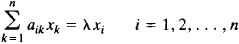# eigenvector

Also found in: Dictionary, Acronyms, Wikipedia.

## eigenvector

[′ī·gən‚vek·tər]
(mathematics)
A nonzero vector v whose direction is not changed by a given linear transformation T ; that is, T (v) = λ v for some scalar λ. Also known as characteristic vector.

## Eigenvector

(or characteristic vector). An eigenvector of a linear transformation is a vector that does not change direction under the transformation and is simply multiplied by a scalar. For example, the eigenvectors of a transformation composed of rotations about some axis and of contraction toward the plane perpendicular to the axis are vectors directed along the axis.

The coordinates x1x2,..., xn of the eigenvectors of a transformation of n-dimensional space with the matrix ║aik║ satisfy the system of homogeneous linear equationswhere λ is an eigenvalue of the matrix. If the matrix of a transformation is Hermitian, then the eigenvectors are mutually perpendicular. As a result of a Hermitian transformation, a sphere becomes an ellipsoid whose major axes are eigenvectors of the transformation.

## eigenvector

(mathematics)
A vector which, when acted on by a particular linear transformation, produces a scalar multiple of the original vector. The scalar in question is called the eigenvalue corresponding to this eigenvector.

It should be noted that "vector" here means "element of a vector space" which can include many mathematical entities. Ordinary vectors are elements of a vector space, and multiplication by a matrix is a linear transformation on them; smooth functions "are vectors", and many partial differential operators are linear transformations on the space of such functions; quantum-mechanical states "are vectors", and observables are linear transformations on the state space.

An important theorem says, roughly, that certain linear transformations have enough eigenvectors that they form a basis of the whole vector states. This is why Fourier analysis works, and why in quantum mechanics every state is a superposition of eigenstates of observables.

An eigenvector is a (representative member of a) fixed point of the map on the projective plane induced by a linear map.
References in periodicals archive ?
In order to maximize the ergodic SNR, the optimized precoded signals to user A should be along the dominant eigenvector of [R.sub.A] namely [v.sub.A] = [u.sub.max]([R.sub.A]).
In this case, only when the eigenvalue [[lambda].sub.i] of the G takes the maximum, the corresponding eigenvector [u.sub.i] is the maximum value.
It shows two ways for how to prove the well-known statement regarding the set of eigenvalues of Aw = [lambda]w with a consistent matrix A of type n x n (n [greater than or equal to] 2), and it demonstrates how to find the corresponding eigenvector components presented as some probabilities of events for maximal eigenvalue [lambda] = [[lambda].sub.max] = n.
Patient 1664 was favoured (based on degree, closeness, betweenness, and eigenvector network centrality metrics) as the most important in the transmission network by having the highest number of secondary cases.
Additionally, we can factor [R.sub.c] to be [R.sub.c] = [U.sub.0][[lambda].sub.c][U.sup.T.sub.0], where [U.sub.0] [member of] [R.sup.nxn] represents a matrix with eigenvectors in a row, while [[LAMBDA].sub.c] represents the diagonal matrix of eigenvalues classified in declining order.
When [S.sub.WT] is nonsingular, the basis vectors [psi] in (3) correspond to the first M([less than or equal to]N) eigenvectors with the largest eigenvalues of [S.sup.-1.sub.WT][S.sub.BT].
In |t> representation, the operator of energy becomes i[??]([partial derivative]/[partial derivative]t), while its eigenvectors |E> become [e.sup.(1/i[??])Et], for every E [member of] R.
In order to speed up the k-means clustering on the embedded eigenvector matrix, we sample row vectors of eigenvectors matrix randomly and get k centers through k-means clustering over the selected row vectors.
The variance of the second to the seventh eigenvector of empirical orthogonal function account for 2.04%, 1.09%, 0.97%, 0.44%, 0.41%, and 0.28%, respectively.

Site: Follow: Share:
Open / Close